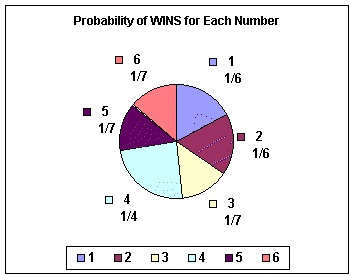#### Probability Homework Help - K-12 Grade Level, College Level Mathematics

Introduction of Probability

The Probability is a measurement of expectation that an event will occur or a statement is true. The Probabilities are given a value, that is in between 0 (will not take place) and 1 (will take place). As the probability of an event is higher, the more certain we are that the event will take place.

The notion has been given an axiomatic mathematical derivation in probability theory that is used broadly in such regions of study as finance, statistics, science, mathematics, artificial intelligence & philosophy, for illustration- draw inferences about the expected frequency of events. The Probability theory is also used to elucidate the underlying mechanics and regularities of the complex systems.Probability theory

The Probability theory is concerned with probability; the examination of arbitrary phenomena. Probability theory is a category of mathematics. The necessary objects of probability theory are stochastic processes, arbitrary variables and events: that is mathematical abstractions of non-deterministic events or measured quantities which might either be single occurrences or change over time in an apparently arbitrary fashion. If the roll of dice or an individual coin toss is considered to be an arbitrary event, then when it is repeated many times the series of random events will display certain patterns that can be predicted and studied. The two representative mathematical outcomes explaining such patterns are the law of large numbers and the central limit theorem.

As a mathematical foundation for statistics, the probability theory is significant to many human activities that include quantitative analysis of large sets of data. Also the methods of probability theory is apply to descriptions of complex systems given only partial knowledge of their condition, as in statistical mechanics. A discovery in 20th century physics was the probabilistic nature of physical phenomena at atomic scales, defined in the quantum mechanics.

Independent probability

If two events, A and B are independent then the joint probability is

P(A and B) = P (A∩B)= P(A)P(B)

#### Mutually exclusive

If either event A or event B or both events occur on a single performance of an experiment this is called the union of the events A and B denoted as. If 2 events are mutually exclusive then the probability of either occurring is

P(A or B) = P (A) = P(A U B)+(B)

#### Not mutually exclusive

If events are not mutually exclusive then

P(A or B)= P (A)+P(B)-P(A and B)

Conditional probability

Conditional probability is the probability of some event A, given the occurrence of other event B. Conditional probability is written, and is read "the probability of A, given B". It is described by

P(A|B)= P(A∩B)/ P (B)

If P(B) =0 is formally undefined by this expression. Though, it is possible to define a conditional probability for some zero-probability events using a σ-algebra of such events (such as those arising from a continuous random variable)

 Summary of probabilities Event Probability A P (A)? [0,1] not A P(AC) = 1- P(A) A or B P(A U B) = P(A)+P(B)-P(A∩B) P(AU B)= P(A)+P(B) if A and B are mutually exclusive A and B P (A∩ B)=P(A|B)P(B)=A(B|A)P(A) P (A∩ B)= P(A)P(B) if A and B are independent A given B P(A|B)= P(A∩B)/ P(B)

Email based Probability Homework Help -Assignment Help

Tutors at the www.tutorsglobe.com are committed to provide the best quality Probability homework help - assignment help. They use their experience, as they have solved thousands of the probability assignments, which may help you to solve your complex Probability homework. You can find solutions for all the topics come under the probability. The dedicated tutors provide eminence work on your Math homework help and devoted to provide K-12 level math to college levelmathshelp before the deadline mentioned by the student. Probability homework help is available here for the students of school, college and university. TutorsGlobe assure for the best quality compliance to your homework. Compromise with quality is not in our dictionary. If we feel that we are not able to provide the homework help as per the deadline or given instruction by the student, we refund the money of the student without any delay.

Qualified and Experienced ProbabilityTutors at www.tutorsglobe.com

Tutors at the www.tutorsglobe.com take pledge to provide full satisfaction and assurance in Probability homework help. Students are getting math homework help services across the globe with 100% satisfaction. We value all our service-users. We provide email based probability homework help - assignment help. You can join us to ask queries 24x7 with live, experienced and qualified math tutors specialized in probability.